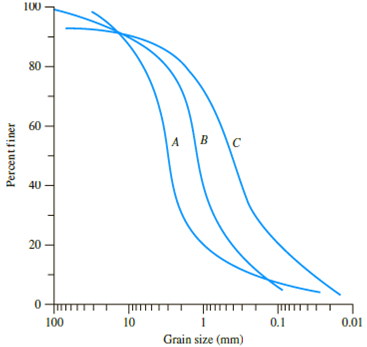Chapter 2, Problem 2.3PPrinciples of Geotechnical Enginee...

9th Edition
Braja M. Das + 1 other
ISBN: 9781305970939

Solutions

Chapter
SectionPrinciples of Geotechnical Enginee...

9th Edition
Braja M. Das + 1 other
ISBN: 9781305970939
Textbook Problem

The grain-size distribution curves for three different sands (A, B, and C) are shown in Figure 2.34.Figure 2.34a. Determine the uniformity coefficient and the coefficient of gradation for each soil.b. Identify if the soils are well graded or poorly graded based on Part a.

(a)

To determine

Calculate the uniformity coefficient (Cu) and coefficient of gradation (Cc) for sands A, B, and C.

Explanation

Sketch the grain size distribution curve for sands A, B, and C as shown in Figure 1.

Refer to Figure 1.

For sand A:

The diameter of the particle corresponding to 60% finer (D60) is 3.5mm.

The diameter of the particle corresponding to 30% finer (D30) is 1.95mm.

The diameter of the particle corresponding to 10% finer (D10) is 0.2mm.

For sand B:

The diameter of the particle corresponding to 60% finer (D60) is 1.5mm.

The diameter of the particle corresponding to 30% finer (D30) is 0.75mm.

The diameter of the particle corresponding to 10% finer (D10) is 0.17mm.

For sand C:

The diameter of the particle corresponding to 60% finer (D60) is 0.55mm.

The diameter of the particle corresponding to 30% finer (D30) is 0.22mm.

The diameter of the particle corresponding to 10% finer (D10) is 0.032mm.

Calculate the uniformity coefficient (Cu) using the relation.

Cu=D60D10 (1)

For sand A:

Substitute 3.5mm for D60 and 0.2mm for D10 in Equation (1).

Cu=3.50.2=17.5

Hence, the uniformity coefficient for sand A is 17.5_.

For sand B:

Substitute 1.5mm for D60 and 0.17mm for D10 in Equation (1).

Cu=1.50.17=8

(b)

To determine

State the sands A, B, and C are well-graded or a poorly-graded.

Still sussing out bartleby?

Check out a sample textbook solution.

See a sample solution

The Solution to Your Study Problems

Bartleby provides explanations to thousands of textbook problems written by our experts, many with advanced degrees!

Get Started

Why is it important to get involved with an engineering organization?

Engineering Fundamentals: An Introduction to Engineering (MindTap Course List)

Describe the Big Data phenomenon.

Database Systems: Design, Implementation, & Management

Why is an object said to have greater semantic content than an entity?

Database Systems: Design, Implementation, & Management

What is the difference between projecting and joining?

Principles of Information Systems (MindTap Course List)

Find state of stress at given point.

Mechanics of Materials (MindTap Course List)

Provide an overview of the systems design phase.

Systems Analysis and Design (Shelly Cashman Series) (MindTap Course List)

Whats the essential difference between an IPS and an IDS?

Network+ Guide to Networks (MindTap Course List)

What should be done with a leaking cylinder if the leak cannot be stopped?

Welding: Principles and Applications (MindTap Course List)